# Poisson Capability HelpThe Poisson capability analysis is used to determine the defects per unit (DPU) meet customer specifications. With the Poisson analysis, the opportunity for defects must be large but the actual number that occur must be small. Each item can more than one defect – it does not mean that the items is defective. So, you use this analysis with counting type attributes data. This analysis has the following output:

• DPU Histogram (with optional target value)
• u Control Chart
• Cumulative DPU Chart
• DPU vs Subgroup Size Chart (if the subgroup size varies)
• P-P Plot (if the subgroup size is constant)
• Statistical Metrics

### Data Entry

The data are entered into a worksheet as shown below (using the first part of the data into the example workbook). The data does not have to start in A1. It can be anywhere on the spreadsheet but must be in columns.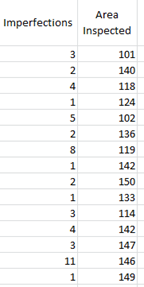In this example, imperfections (defects) in a plastic sheet are being tracked. The data are collected for each batch. The first column is the number of imperfections (c) in a sheet. The second column is the subgroup size – the area of the plastic sheet (n). If there is a constant subgroup size, this range is not needed. You will enter the subgroup size below. The columns for c and n do not have to be together.

### Creating a Poisson Capability Analysis

1. Select the data on the worksheet to be included in the analysis as shown by the shaded area above. You can also just put the cursor in the first cell of the data. The program will automatically select the data underneath that cell.

2. Select “Poisson: from the “Process Capability” panel on the SPC for Excel ribbon.

3. The Poisson Capability form below shown.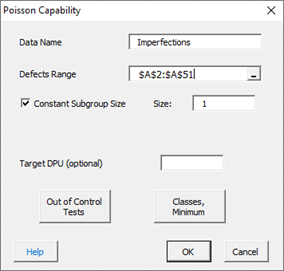• Data Name: the name of the data, e.g., “Imperfections”, this is optional
• Defects Range: the range of the number of defects you can edit the range if needed here.
• Constant Subgroup Size: default is yes, use a constant subgroup size.
• Size: Enter the constant subgroup size if that option is selected, default is 1
• Subgroup Size Range: the range of the subgroups if the size varies; this appears when the Constant Subgroup Size checkbox is unchecked; you can edit the range if needed here.
• Target DPU (optional): the target value for DPU
• Out of Control Tests: options to select which out of control tests to apply to the u chart
• Classes, Minimum: options for the histogram
• Select OK to create the Poisson Capability Analysis
• Select Cancel to exit the program

If you uncheck the “Constant Subgroup Size” box in the form above, the subgroup range becomes visible as shown below: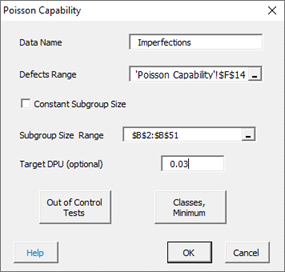The output is shown below.  A target of 0.03 was used for DPU.

### Output for the Poisson Capability Analysis

The output from the analysis is placed a new worksheet. There are five parts to the output.

Statistical Metrics

The part of the output provides the statistical metrics from the analysis including average DPU and confidence interval associated with that average.
Histogram

The histogram shows the distribution of DPU values. If you entered a target values, it is plotted on the histogram to allow you to assess if most of the subgroups meet that target or not.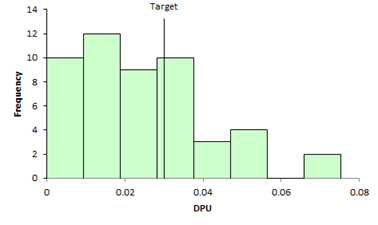u Control Chart

The u control chart assesses the state of statistical control of the results. The center line is the average DPU. The two dotted lines are the upper and lower control points. The process is in statistical control if none of the selected control chart test above are violated. If the p control chart is in statistical control, the results are assumed to be valid. If there are too many out of control points, the process is not stable, and the results are not valid. Out of control points are in red.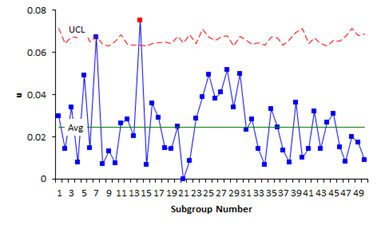Cumulative DPU Chart

This chart plots the cumulative DPU over time for each subgroup. It is used to determine if you have enough subgroups for a valid estimate of the average DPU. The center line is the average DPU (same as the center line on the u control chart). The upper and lower confidence limits for the average DPU are also plotted. You want the data to flatten out along the average DPU line. If this occurs, you have enough subgroups.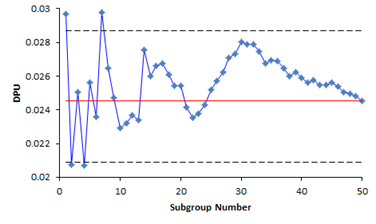DPU vs Subgroup Size Chart
If the subgroup size varies, a DPU vs subgroup size chart is included in the output. This chart plots DPU for each subgroup size against the subgroup size. It is used to help determine if the data comes from a binomial distribution. If it does, you would expect the points to be randomly distributed around the centerline, which is the average DPU.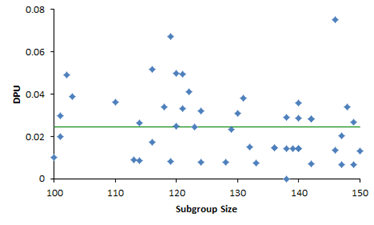P-P Plot

If the subgroup size is constant, a P-P Plot is included in the output. This P-P Plot is used to determine if the data comes from a Poisson distribution. The P-P Plot plots the empirical cumulative distribution function (CDF) values (based on the data) against the theoretical CDF values (based on the Poisson distribution). If the P-P plot is close to a straight line, then the Poisson distribution fits the data.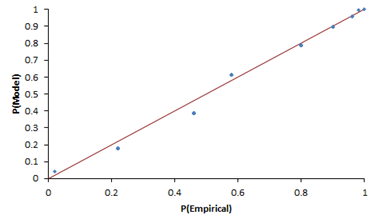### Options for the Poisson Capability Analysis

There are two options on the input form shown above: Out of Control Tests and Classes, Minimum. Both are described below.

Out of Control Tests

Selecting this option displays the input screen below. These options apply to the p control chart which is used in the Poisson Capability Analysis. This defines what out of control tests to apply to the control chart.

• Point beyond the control limits: out of control if a point that is above the upper control or below the lower control
• k points in a row trending up or down: out of control if there are k points in a row trending upward or trending downward; two points in a row that are equal break this trend
• k points in a row in Zone C or beyond (one side of avg): Zone C is the zone between the average and one standard deviation above the average on the top half of the chart and the average and one standard deviation below the average on the bottom half of the chart; out of control if there is a run of k points in a row above or below the average
• Overcontrol: k points in row alternating up and down: out of control if k points in a row are such that the values alternate up and down

Classes, Minimum

If you select the “Classes, Minimum” option, the following input screen will appear:

• Enter number of classes: this option sets the number of classes (bars) on the histogram
• Enter class width: this option changes the width of the classes (bars)
• Enter lower class boundary: this option sets the lower boundary on the histogram
• Select OK to use selected options
• Select Cancel to revert to previous options